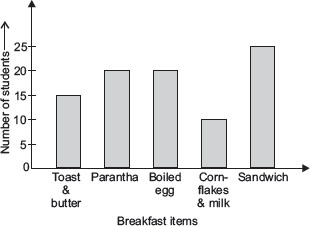# User ForumSubject :IMO    Class : Class 5

The given bar graph shows the favourite breakfast items of the students of Class V. Read the bar graph and answer the questions.How many students gave their choices?

A 65
B 85
C 80
D 90

I calculated it but it came 85

## Ans 1:

Class : Class 5
When i calculated I got 90.

## Ans 2:

Class : Class 6
You all are explicabally right

## Ans 3:

Class : Class 6
yes, the answer is 90 (d)

Class : Class 7
The answer is D

## Ans 5:

Class : Class 5
correct answer is D

## Ans 6:

Class : Class 6
Ans is D. Think like this:[(15+20)+(20+10)]+25=(35+30)+25=65+25=90.

Class : Class 5
ThanQ friends

Class : Class 6